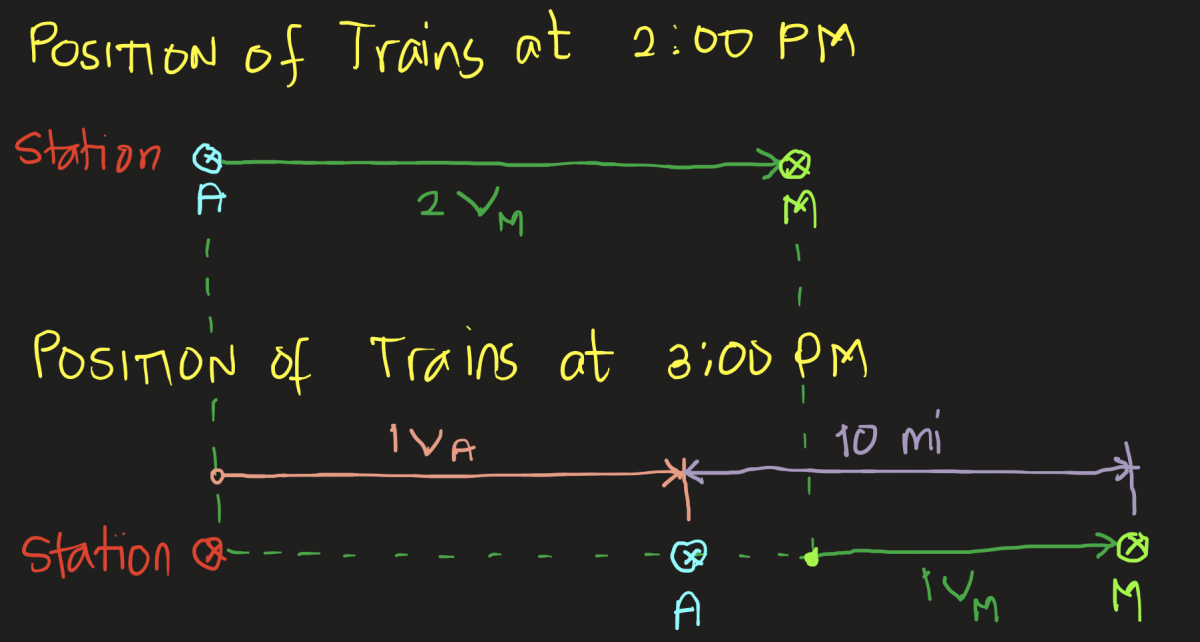# Physics: Uniform Motion

A Metra commuter train leaves
Union Station in Chicago at 12 noon. Two hours later, an
Amtrak train leaves on the same track, traveling at an
average speed that is 50 miles per hour faster than the
Metra train. At 3 PM the Amtrak train is 10 miles behind the
commuter train. How fast is each going?

Tags:### Consider the figure below:

Consider the figure below:Given: $v_A = v_M + 50$

And from the figure:
$3v_M = v_A + 10$

$v_M = 30 ~ \text{mph}$

$v_A = 80 ~ \text{mph}$

### sir anong gamit mo paggawa ng

sir anong gamit mo paggawa ng figure?### Microsoft One Note

For the screenshot above, Microsoft OneNote.

• Mathematics inside the configured delimiters is rendered by MathJax. The default math delimiters are $$...$$ and $...$ for displayed mathematics, and $...$ and $...$ for in-line mathematics.